The Derivative and Tangent Line Problem

The use of differentiation makes it possible to solve the tangent line problem by finding the slope $f'(a)$.

Learning Objectives

Define a derivative as the slope of the tangent line to a point on a curve

Key Takeaways

Key Points

• The tangent line $t$ (or simply the tangent) to a plane curve at a given point is the straight line that “just touches” the curve at that point.
• A straight line is said to be a tangent of a curve $y=f(x)$ at a point $x = c$ on the curve if the line passes through the point $(c, f(c))$ on the curve and has slope $f'(c)$ where $f'$ is the derivative of $f$.
• Using derivatives, the equation of the tangent line can be stated as follows: $y = f(a) + f{(a)}'(x-a)$.

Key Terms

• tangent: a line touching a curve at a single point without crossing it there
• secant: a line that intersects a curve at two or more points

The tangent line $t$ (or simply the tangent) to a plane curve at a given point is the straight line that “just touches” the curve at that point. Informally, it is a line through a pair of infinitely close points on the curve. More precisely, a straight line is said to be a tangent of a curve $y = f(x)$ at a point $x = c$ on the curve if the line passes through the point $(c, f(c))$ on the curve and has slope $f'(c)$ where f‘ is the derivative of $f$.Tangent to a Curve: The line shows the tangent to the curve at the point represented by the dot. It barely touches the curve and shows the rate of change slope at the point.

Suppose that a curve is given as the graph of a function, $y = f(x)$. To find the tangent line at the point $p = (a, f(a))$, consider another nearby point $q = (a + h, f(a + h))$ on the curve. The slope of the secant line passing through $p$ and $q$ is equal to the difference quotient

$\displaystyle{\frac{f(a + h) - f(a)}{h}}$.

As the point $q$ approaches $p$, which corresponds to making $h$ smaller and smaller, the difference quotient should approach a certain limiting value $k$, which is the slope of the tangent line at the point $p$. If $k$ is known, the equation of the tangent line can be found in the point-slope form:

$y - f(a) = k(x-a)$

Suppose that the graph does not have a break or a sharp edge at $p$ and it is neither vertical nor too wiggly near $p$. Then there is a unique value of $k$ such that, as $h$approaches $0$, the difference quotient gets closer and closer to $k$, and the distance between them becomes negligible compared with the size of $h$, if $h$ is small enough. This leads to the definition of the slope of the tangent line to the graph as the limit of the difference quotients for the function $f$. This limit is the derivative of the function $f$ at $x=a$, denoted $f'(a)$. Using derivatives, the equation of the tangent line can be stated as follows:

$y = f(a) + f{(a)}'(x-a)$.

Derivatives and Rates of Change

Differentiation is a way to calculate the rate of change of one variable with respect to another.

Learning Objectives

Describe the derivative as the change in $y$ over the change in $x$ at each point on a graph

Key Takeaways

Key Points

• Historically, the primary motivation for the study of differentiation was the tangent line problem, which is the task of, for a given curve, finding the slope of the straight line that is tangent to that curve at a given point.
• If $y$ is a linear function of $x$, then $m = \frac{\Delta y}{\Delta x}$.
• The derivative measures the slope of a graph at each point.

Key Terms

• slope: also called gradient; slope or gradient of a line describes its steepness

Historically, the primary motivation for the study of differentiation was the tangent line problem, which is the task of, for a given curve, finding the slope of the straight line that is tangent to that curve at a given point. The word tangent comes from the Latin word tangens, which means touching. Thus, to solve the tangent line problem, we need to find the slope of a line that is “touching” a given curve at a given point, or, in modern language, that has the same slope. But what exactly do we mean by “slope” for a curve?

The simplest case is when $y$ is a linear function of x, meaning that the graph of $y$ divided by $x$ is a straight line. In this case, $y = f(x) = m x + b$, for real numbers m and b, and the slope m is given by:

$\displaystyle{m = \frac{\Delta y}{\Delta x}}$

where the symbol $\Delta$ (the uppercase form of the Greek letter Delta) means, and is pronounced, “change in.” This formula is true because:

$y + \Delta y = f(x+ \Delta x) = m (x + \Delta x) + b = m x + b + m \Delta x = y + m\Delta x$

It follows that $\Delta y = m \Delta x$.Slope of a function: A function with the slope shown for a given point.

This gives an exact value for the slope of a straight line. If the function $f$ is not linear (i.e., its graph is not a straight line), however, then the change in $y$ divided by the change in $x$ varies: differentiation is a method to find an exact value for this rate of change at any given value of $x$. In other words, differentiation is a method to compute the rate at which a dependent output $y$ changes with respect to the change in the independent input $x$. This rate of change is called the derivative of $y$ with respect to $x$. In more precise language, the dependence of $y$ upon $x$ means that $y$ is a function of $x$. This functional relationship is often denoted $y=f(x)$, where $f$ denotes the function. If $x$ and $y$ are real numbers, and if the graph of $y$ is plotted against $x$, the derivative measures the slope of this graph at each point.

The Derivative as a Function

If every point of a function has a derivative, there is a derivative function sending the point $a$ to the derivative of $f$ at $x = a$: $f'(a)$.

Learning Objectives

Explain how the derivative acts as a “function of functions”

Key Takeaways

Key Points

• The derivative is an operator whose domain is the set of all functions that have derivatives at every point of their domain and whose range is a set of functions.
• The function whose value at $x=a$ equals $f′(a)$ whenever $f′(a)$ is defined and elsewhere is undefined is also called the derivative of $f$.
• By the definition of the derivative function, $D(f)(a) = f′(a)$, where $D$ is an operator whose domain is the set of all functions that have derivatives at every point of their domain and whose range is a set of functions.

Key Terms

• range: the set of values (points) which a function can obtain
• domain: the set of all possible mathematical entities (points) where a given function is defined

Let $f$ be a function that has a derivative at every point $a$ in the domain of $f$. Because every point $a$ has a derivative, there is a function that sends the point $a$ to the derivative of $f$ at $a$. This function is written $f'(x)$ and is called the derivative function or the derivative of $f$. The derivative of $f$ collects all the derivatives of $f$ at all the points in the domain of $f$. Visually, derivative of a function $f$ at $x=a$ represents the slope of the curve at the point $x=a$.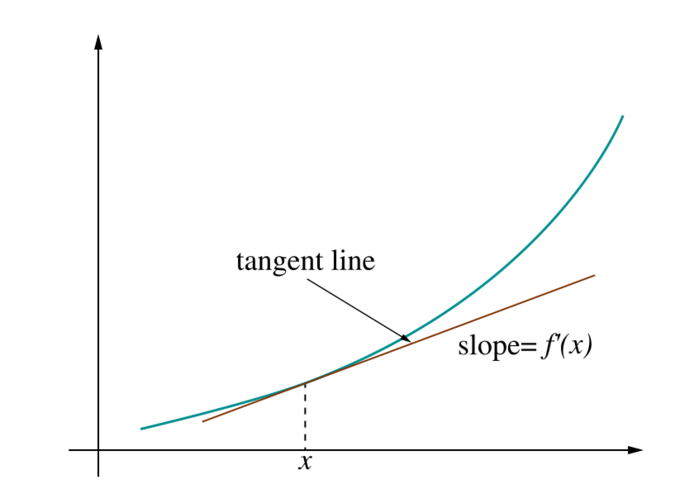Derivative As Slope: The slope of tangent line shown represents the value of the derivative of the curved function at the point $x$.

Sometimes $f$ has a derivative at most, but not all, points of its domain. The function whose value at $a$ equals $f'(a)$ whenever $f'(a)$ is defined and elsewhere is undefined is also called the derivative of $f$. It is still a function, but its domain is strictly smaller than the domain of $f$.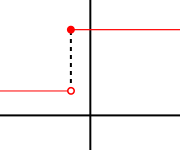Discontinuous Function: At the point where the function makes a jump, the derivative of the function does not exist.

Using this idea, differentiation becomes a function of functions: The derivative is an operator whose domain is the set of all functions that have derivatives at every point of their domain and whose range is a set of functions. If we denote this operator by $D$, then $D(f)$ is the function $f'(x)$. Since $D(f)$ is a function, it can be evaluated at a point $a$. By the definition of the derivative function, $D(f)(a)=f'(a)$.

For comparison, consider the doubling function $f(x) = 2x$; $f$ is a real-valued function of a real number, meaning that it takes numbers as inputs and has numbers as outputs:

$1 \rightarrow 2$

$2 \rightarrow 4$

$3 \rightarrow 6$.

The operator $D$, however, is not defined on individual numbers. It is only defined on functions:

$D(x \rightarrow 1) = (x \rightarrow 0)$

$D(x \rightarrow x) = (x \rightarrow 1)$

$D(x \rightarrow x^2) = (x \rightarrow 2x)$.

Because the output of $D$ is a function, the output of $D$ can be evaluated at a point. For instance, when $D$ is applied to the squaring function,

$x \rightarrow x^2$

$D$ outputs the doubling function,

$x \rightarrow 2x$

which is f(x)f(x). This output function can then be evaluated to get $f(1)=2f(1) = 2$, $f(2) = 4$, and so on.

Differentiation Rules

The rules of differentiation can simplify derivatives by eliminating the need for complicated limit calculations.

Learning Objectives

Practice using differentiation rules to simplify differentiating complicated expressions

Key Takeaways

Key Points

• Differentiation by polynomial expansion can be very complicated and prone to errors.
• Constant rule: if $f(x)$ is a constant, then its derivative, $f'(x)$, is $0$.
• Chain Rule: If $f(x) = h(g(x))$, then $f'(x) = h'(g(x)) g'(x)$.
• Product Rule: $(fg)' = f'g + g'f$.
• Quotient Rule: $\left ( \frac {f}{g} \right )' = \frac {f'g - fg'}{g^2}$.

Key Terms

• difference quotient: the function difference $\Delta F$ divided by the point difference $\Delta x$: $\Delta F(x) / \Delta x$
• polynomial: an expression consisting of a sum of a finite number of terms, each term being the product of a constant coefficient and one or more variables raised to a non-negative integer power

When we wish to differentiate complicated expressions, a possible way to differentiate the expression is to expand it and get a polynomial, and then differentiate that polynomial. This method becomes very complicated and is particularly error prone when doing calculations by hand. In many cases, complicated limit calculations by direct application of Newton’s difference quotient can be avoided by using differentiation rules. Some of the most basic rules are the following.

The Constant Rule

If $f(x)$ is a constant, then $f'(x) = 0$, since the rate of change of a constant is always zero.

The Sum Rule

$(\alpha f + \beta g)' = \alpha f' + \beta g'$

for all functions $f$ and $g$ and all real numbers $\alpha$ and $\beta$.

The Product Rule

$(fg)' = f'g + g'f$

for all functions $f$ and $g$.

By extension, this means that the derivative of a constant times a function is the constant times the derivative of the function.

The Quotient Rule

$\displaystyle{\left ( \frac {f}{g} \right )' = \frac {f'g - fg'}{g^2}}$

for all functions $f$ and $g$ at all inputs where $g \neq 0$.Model Rockets: The flight of model rockets can be modeled using the product rule.

The Chain Rule

If $f(x) = h(g(x))$, then $f'(x) = h'(g(x)) g'(x)$.

Example

Consider the following function:

$f(x) = x^4 + e^{x^2} - ln(x)e^x + 7$

Differentiating yields:

$\displaystyle{f'(x) = 4x^{(4-1)} + \frac{d(x^2)}{dx}e^{x^2} - \frac {d(ln\:x)}{dx}e^x - ln\:x\frac{d(e^x)}{dx} + 0 \\ \qquad = 4x^3 + 2xe^{x^2} - \frac {1}{x}e^x - ln(x)e^x}$

Here the second term was computed using the chain rule and the third using the product rule. The known derivatives of the elementary functions $x^2$, $x^4$, $\ln(x)$, and $e^x$, as well as that of the constant 7, were also used.

Derivatives of Trigonometric Functions

Derivatives of trigonometric functions can be found using the standard derivative formula.

Learning Objectives

Identify the derivatives of the most common trigonometric functions

Key Takeaways

Key Points

• The derivative of the sine function is the cosine function.
• The derivative of the cosine function is the negative of the sine function.
• The derivative of the tangent function is the squared secant function.

Key Terms

• secant: a straight line that intersects a curve at two or more points

The trigonometric functions (also called the circular functions) are functions of an angle. They are used to relate the angles of a triangle to the lengths of the sides of a triangle. Trigonometric functions are important in the study of triangles and modeling periodic phenomena, among many other applications.

The most familiar trigonometric functions are the sine, cosine, and tangent. In the context of the standard unit circle with radius 1, where a triangle is formed by a ray originating at the origin and making some angle with the $x$-axis, the sine of the angle gives the length of the $y$-component (rise) of the triangle, the cosine gives the length of the $x$-component (run), and the tangent function gives the slope ($y$-component divided by the $x$-component).

With this in mind, we can use the definition of a derivative to calculate the derivatives of different trigonometric functions:

$\displaystyle{f'(x) = \lim_{h\rightarrow 0} \frac {f(x+h) - f(x)}{h}}$

For example, if $f(x) = \sin x$, then:

$\displaystyle{f'(x) = \lim_{h\rightarrow 0} \frac {\sin (x+h) - \sin (x)}{h}}$

$\displaystyle{ \quad \quad = \lim_{h\rightarrow 0} \frac{\cos(x) \cdot \sin(h) + \cos(h) \cdot \sin(x) - \sin(x)}{h}}$

$\displaystyle{ \quad \quad = \lim_{h\rightarrow 0} \frac{\cos(x) \cdot \sin(h) + (\cos(h) - 1) \cdot \sin(x)}{h}}$

$\displaystyle{ \quad \quad = \lim_{h\rightarrow 0} \frac{\cos(x) \cdot \sin(h)}{h} + \lim_{h\rightarrow 0} \frac {(\cos(h) - 1) \cdot \sin(x)}{h}}$

$\displaystyle{ \quad \quad = \cos (x) (1) + \sin (x) (0)}$

$\displaystyle{ \quad \quad = \cos (x)}$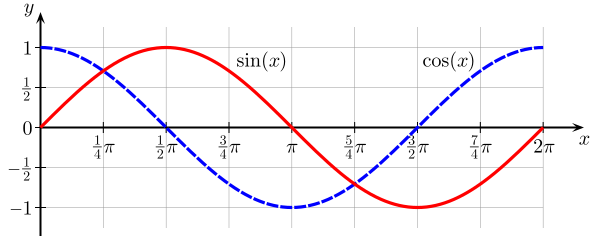Sine and Cosine: In this image, one can see that where the line tangent to one curve has zero slope (the derivative of that curve is zero), the value of the other function is zero.

The same procedure can be applied to find other derivatives of trigonometric functions. The most common are the following:

$\displaystyle{\frac{d}{dx} \cos x = -\sin x}$

$\displaystyle{\frac{d}{dx} \tan x = \sec^2 x}$

The Chain Rule

The chain rule is a formula for computing the derivative of the composition of two or more functions.

Learning Objectives

Calculate the derivative of a composition of functions using the chain rule

Key Takeaways

Key Points

• If $f$ is a function and $g$ is a function, then the chain rule expresses the derivative of the composite function $f \circ g$ in terms of the derivatives of $f$ and $g$.
• The chain rule can be applied sequentially for as many functions as are nested inside one another.
• The chain rule for $f \circ g(x)$ is $\frac{df}{dx} = \frac{df}{dg}\frac{dg}{dx}$.

Key Terms

• composite: a function of a function

The chain rule is a formula for computing the derivative of the composition of two or more functions. That is, if $f$ is a function and $g$ is a function, then the chain rule expresses the derivative of the composite function $f \circ g$ in terms of the derivatives of $f$ and $g$.

For example, following the chain rule for $f \circ g(x) = f[g(x)]$ yields:

$\displaystyle{\frac{df}{dx} = \frac{df}{dg} \cdot \frac{dg}{dx}}$

The method is called the “chain rule” because it can be applied sequentially to as many functions as are nested inside one another. For example, if $f$ is a function of $g$, which is in turn a function of $h$, which is in turn a function of $x$—that is, $f(g(h(x)))$—then the derivative of $f$ with respect to $x$ is:

$\displaystyle{\frac{df}{dx} = \frac{df}{dg} \cdot \frac{dg}{dh} \cdot \frac{dh}{dx}}$

The chain rule has broad applications in physics, chemistry, and engineering, as well as for the study of related rates in many other disciplines. The chain rule can also be generalized to multiple variables in cases where the nested functions depend on more than one variable.Skydiving: The path of a skydiver relies on many variables such as time and height. Use of the chain rule is needed for the complicated calculation.

Consider the function $f(x) = (x^2 + 1)^3$. Using the chain rule yields:

$f(x) = (x^2 + 1)^3$

$u(x) = x^2 + 1$

$f(x) = [u(x)]^3$

Substituting $f(u)$ and $u(x)$, we get

$\displaystyle{\frac {df}{dx} = \frac {df}{du} \cdot \frac {du}{dx}}$

$\displaystyle{ \qquad = \frac {d}{du}u^3 \frac {d}{dx} (x^2 + 1)}$

$\quad \quad = (3u^2) (2x)$

$\quad \quad = \left ( 3(x^2 + 1)^2 \right ) \cdot (2x)$

$\quad \quad = 6x(x^2 + 1)^2$

Implicit Differentiation

Implicit differentiation makes use of the chain rule to differentiate implicitly defined functions.

Learning Objectives

Use implicit differentiation to find the derivatives of functions that are not explicitly functions of $x$

Key Takeaways

Key Points

• As y can be given as a function of $x$ implicitly rather than explicitly, when we have an equation $R(x, y) = 0$, we may be able to solve it for $y$ and then differentiate.
• An implicit function is a function that is defined implicitly by a relation between its argument and its value.
• The implicit function theorem states that if the left-hand side of the equation $R(x, y) = 0$ is differentiable and satisfies some mild condition on its partial derivatives at some point $(a, b)$ such that $R(a, b) = 0$, then it defines a function $y = f(x)$ over some interval containing $a$.

Key Terms

• implicit: implied indirectly, without being directly expressed

An implicit function is a function that is defined implicitly by a relation between its argument and its value.

If the left hand side of the equation $R(x, y) = 0$ is differentiable and satisfies some mild condition on its partial derivatives at some point $(a, b)$ such that $R(a, b) = 0$, then it defines a function $y = f(x)$ over some interval containing $a$. Geometrically, the graph defined by $R(x, y) = 0$ will overlap locally with the graph of some equation $y = f(x)$.

For most implicit functions, there is no formula which defines them explicitly. However, various numerical methods exist for computing approximately the value of $y$ corresponding to any fixed value of $x$; this allows us to find an explicit approximation to the implicit function.

Implicit differentiation makes use of the chain rule to differentiate implicitly defined functions.

Previously, the functions we have investigated were explicit functions of one variable with respect to another. $y= x^2$ is an explicit function because it shows you exactly what $y$ is with respect to $x$. An implicit function doesn’t tell you exactly what $y$ is but still relates $y$ to $x$. For example, $y^2+y=\sin (x)$ is an implicit function because it is very difficult to get a formula for $y$ in terms of only $x$. However, we can still find the derivative of $y$ with respect to $x$ by using implicit differentiation.

$\displaystyle{2y \cdot \frac{dy}{dx}+\frac{dy}{dx} =\cos(x)=(2y+1)\frac{dy}{dx}}$

Therefore:

$\displaystyle{\frac{dy}{dx}=\frac{\cos(x)}{2y+1}}$

As $y$ can be given as a function of $x$ implicitly rather than explicitly, when we have an equation $R(x, y) = 0$, we may be able to solve it for $y$ and then differentiate. However, sometimes it is simpler to differentiate $R(x, y)$ with respect to $x$ and $y$ and then solve for $\frac{dy}{dx}$.

For example, given the expression $y + x + 5 = 0$, differentiating yields:

$\displaystyle{\frac {dy}{dx} + \frac{dx}{dx} + \frac {d}{dx}5 = \frac {dy}{x} + 1 = 0}$.

Solving for $\frac{dy}{dx}$ yields:

$\displaystyle{\frac {dy}{dx} = -1}$

A circle can be described by the equation $x^2+y^2=r^2$ where $r$ is the radius of the circle. You can use implicit differentiation to find the slope of a line tangent to the circle at a point $(x,y)$. Since the slope of a tangent is the derivative at that point, we find the derivative implicitly:

$\displaystyle{2y \frac{dy}{dx} +2x \frac{dx}{dx}=\frac{dr}{dx}=0}$

where $\frac{dr}{dx}$ is $0$ since the radius is constant. You can then find a formula for $\frac{dy}{dx}$:

$\displaystyle{\frac{dy}{dx}=\frac{-2x}{2y}=\frac{-x}{y}}$

and you can now find the slope at any point $(x,y)$.Path of a Point on a Circle: The path of a point on a circle can only be expressed as an implicit function.

Differentiation and Rates of Change in the Natural and Social Sciences

Differentiation, in essence calculating the rate of change, is important in all quantitative sciences.

Learning Objectives

Give examples of differentiation, or rates of change, being used in a variety of academic disciplines

Key Takeaways

Key Points

• Differentiation has applications to nearly all quantitative disciplines, whether it’s natural or social science.
• Physical scientists use differentiation and rate of change to study the way a physical concept changes over time or distance.
• Social scientists use differentiation and rate to determine how people, goods, and processes change due to the change of an independent variable.

Key Terms

• differential geometry: the study of geometry using differential calculus
• momentum: (of a body in motion) the product of its mass and velocity
• gross domestic product: a measure of the economic production of a particular territory in financial capital terms over a specific time period

Given a function $y=f(x)$, differentiation is a method for computing the rate at which a dependent output $y$ changes with respect to the change in the independent input $x$. This rate of change is called the derivative of $y$ with respect to $x$.

Rate of change is an important concept in many quantitative studies, and it is no surprise that differentiation (representing the rate of change) has applications to nearly all quantitative disciplines. For example, in physics, the derivative of the displacement of a moving body with respect to time is the velocity of the body, and the derivative of velocity with respect to time is acceleration. Newton’s second law of motion states that the derivative of the momentum of a body equals the force applied to the body. The reaction rate of a chemical reaction is a derivative. In operations research, derivatives determine the most efficient ways to transport materials and design factories.

Derivatives are frequently used to find the maxima and minima of a function. Equations involving derivatives are called differential equations and are fundamental in describing natural phenomena. Derivatives and their generalizations appear in many fields of mathematics, such as complex analysis, functional analysis, differential geometry, measure theory and abstract algebra.

Rates of change occur in all sciences and across all disciplines. Economists study the rate of change of gross domestic product and social scientists the rate in which populations vote in a specific area. Geologists study the rate of earth shift and the temperature gradient of rocks near a volcano. Accountants study the rate of change of production and supplies, and how any change can affect cost and profit. Urban engineers study the flow of traffic in order to design and build more efficient roads and freeways.

In every aspect of life in which something changes, differentiation and rates of change are an important aspect in understanding the world and finding ways to improve it.

Related Rates

Related rates problems involve finding a rate by relating that quantity to other quantities whose rates of change are known.

Learning Objectives

Solve problems using related rates (using a quantity whose rate is known to find the rate at which a related quantity changes)

Key Takeaways

Key Points

• Because science and engineering often relate quantities to each other, the methods of related rates have broad applications in these fields.
• Because problems involve several variables, differentiation with respect to time or one of the other variables requires application of the chain rule.
• The process for solving related rates problems: Write out any relevant formulas and information, take the derivative of the primary equation with respect to time, solve for the desired variable, plug in known information and simplify.

Key Terms

• variable: a quantity that may assume any one of a set of values

One useful application of derivatives is as an aid in the calculation of related rates. What is a related rate? In differential calculus, related rates problems involve finding a rate at which a quantity changes by relating that quantity to other quantities whose rates of change are known. In most cases, the related rate that is being calculated is a derivative with respect to some value. We compute this derivative from a rate at which some other known quantity is changing. Given the rate at which something is changing, we are asked to find the rate at which a value related to the rate we are given is changing.

The rate of change is usually with respect to time. Because science and engineering often relate quantities to each other, the methods of related rates have broad applications in these fields. Because problems involve several variables, differentiation with respect to time or one of the other variables requires application of the chain rule.

Process for solving related rates problems:

1. Write out any relevant formulas and information.

2. Take the derivative of the primary equation with respect to time.

3. Solve for the desired variable.

4. Plug in known information and simplify.

See for a flowchart for solving related rates problems.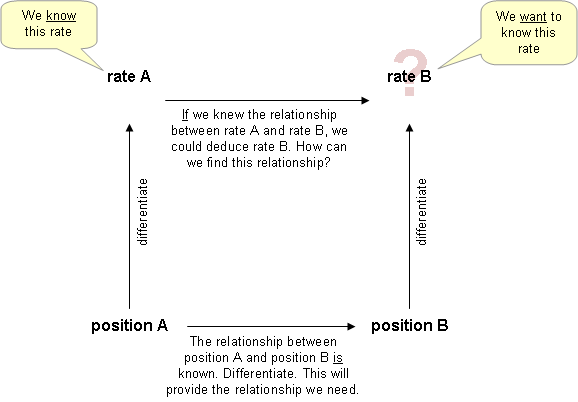Flow Chart for Related Rate Problem Solving: Related rate problems cab be handled by taking a methodical approach.

Suppose you are given the following situation.

A spherical hot air balloon is being filled with air. The volume is changing at a rate of 2 cubic feet per minute. How is the radius changing with respect to time when the radius is equal to 2 feet?

The relevant formulas and pieces of information are the volume of the balloon, the rate of change of the volume, and the radius.

$\displaystyle{V = \frac{4}{3}\pi r^3}$

$V' = 2$

$r = 2$.

Take the derivative of both sides with respect to time:

$\displaystyle{V' = \frac{4}{3}(3)\pi r^2 r'}$

$\,\, \quad = {4}\pi r^2 r'$

Solve for $r$:

$\displaystyle{r ‘ = \frac{1}{4\pi r^2}V'}$

Plug in the known information:

$\displaystyle{r' = \frac {1}{16 \pi}2 = \frac {1}{8 \pi}}$ ft/min

Higher Derivatives

The derivative of an already-differentiated expression is called a higher-order derivative.

Learning Objectives

Compute higher (second, third, etc.) derivatives of functions

Key Takeaways

Key Points

• The second derivative, or second order derivative, is the derivative of the derivative of a function.
• Because the derivative of a function is defined as a function representing the slope of the original function, the double derivative is the function representing the slope of the first-derivative function.
• If $x(t)$ represents the position of an object at time $t$, then the higher-order derivatives of $x$ have physical interpretations, such as velocity and acceleration.

Key Terms

• derivative: a measure of how a function changes as its input changes

The second derivative, or second order derivative, is the derivative of the derivative of a function. The derivative of the function may be denoted by $f'(x)$, and its double (or “second”) derivative is denoted by $f''(x)$. This is read as “$f$ double prime of $x$,” or “the second derivative of $f(x)$. ” Because the derivative of a function is defined as a function representing the slope of function, the double derivative is the function representing the slope of the first derivative function.

Furthermore, the third derivative is the derivative of the derivative of the derivative of a function, which can be represented by $f'''(x)$. This is read as “$f$ triple prime of $x$“, or “the third derivative of $f(x)$.”. This can continue as long as the resulting derivative is itself differentiable, with the fourth derivative, the fifth derivative, and so on. Any derivative beyond the first derivative can be referred to as a higher order derivative.

If $x(t)$ represents the position of an object at time $t$, then the higher-order derivatives of $x$ have physical interpretations. The second derivative of $x$ is the derivative of $x'(t)$, the velocity, and by definition is the object’s acceleration. The third derivative of $x$ is defined to be the jerk, and the fourth derivative is defined to be the jounce. A function $f$ need not have a derivative—for example, if it is not continuous. Similarly, even if $f$ does have a derivative, it may not have a second derivative. See for a graphical illustration of higher derivatives in physics.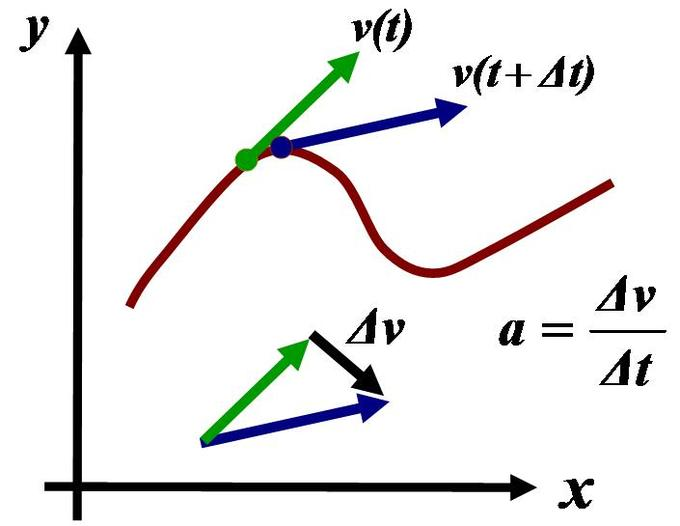Acceleration: Acceleration is the time-rate of change of velocity, and the second-order rate of change of position.

An example of a function with higher-order derivatives is:

$f(x) = 5x^3 + 3x^2 - x + 4$

where the higher derivatives are found to be:

• $f'(x) = 15x^2 + 6x - 1$
• $f''(x) = 30x + 6$
• $f'''(x) = 30$